Ex 7.6

Chapter 7 Class 12 Integrals
Serial order wise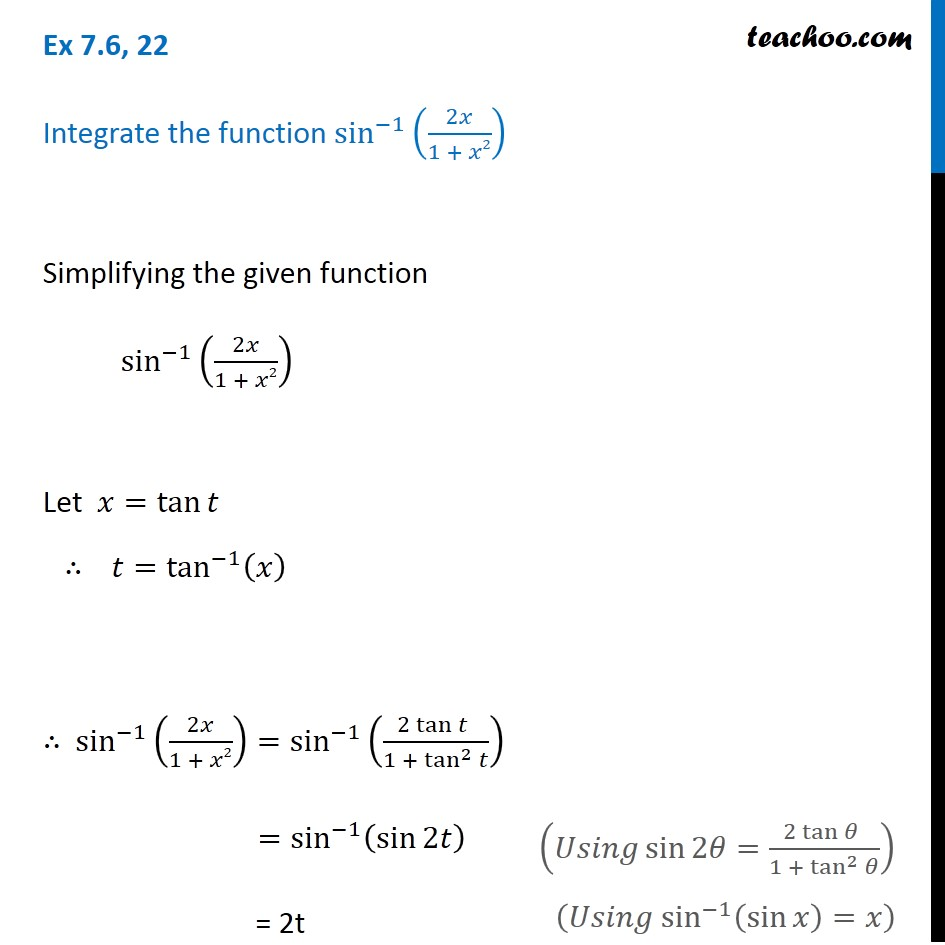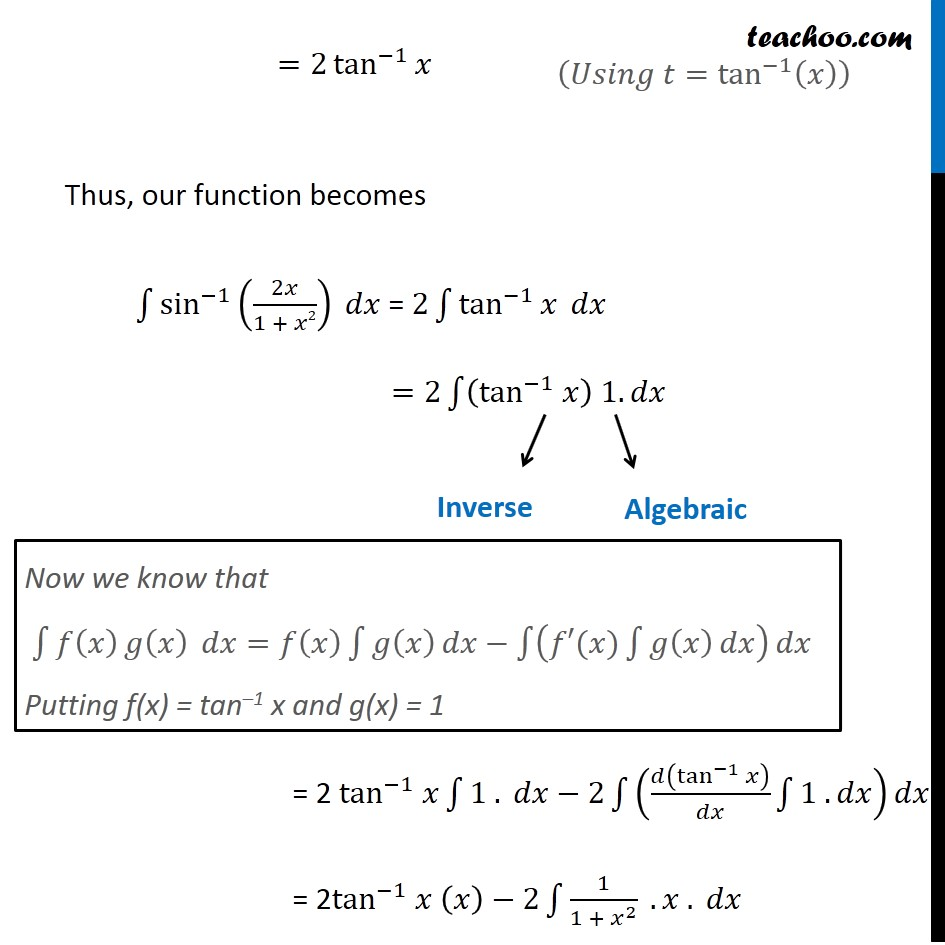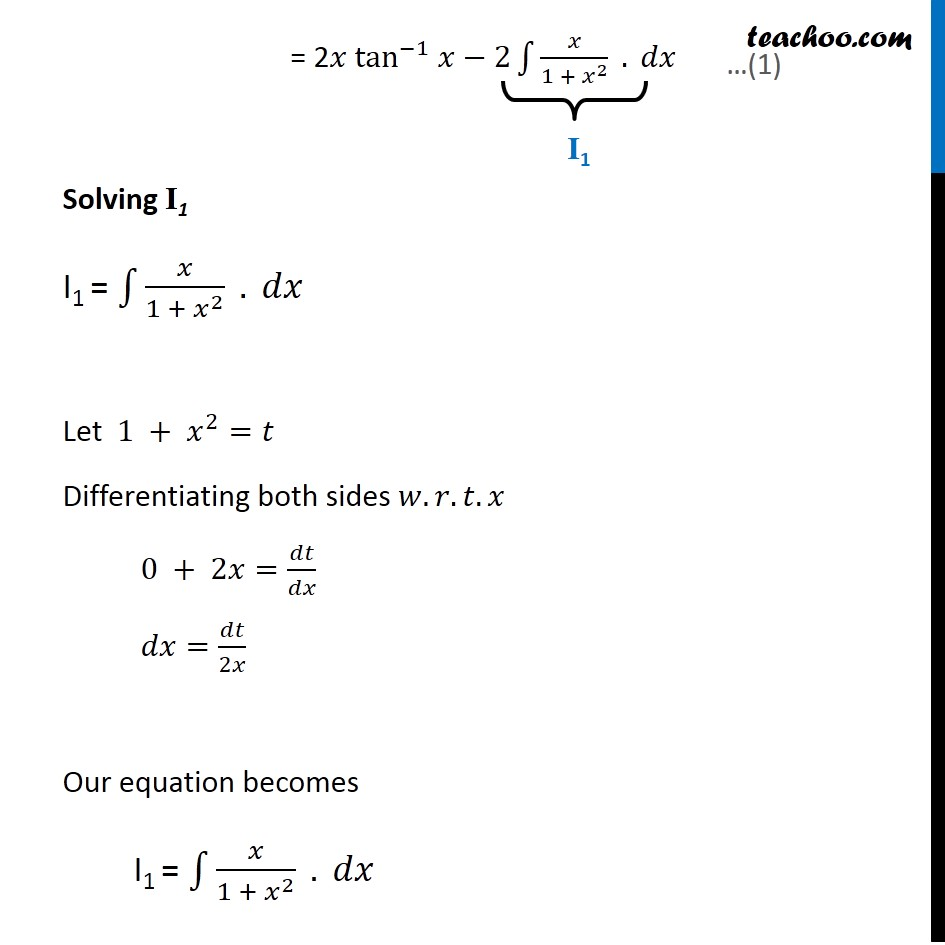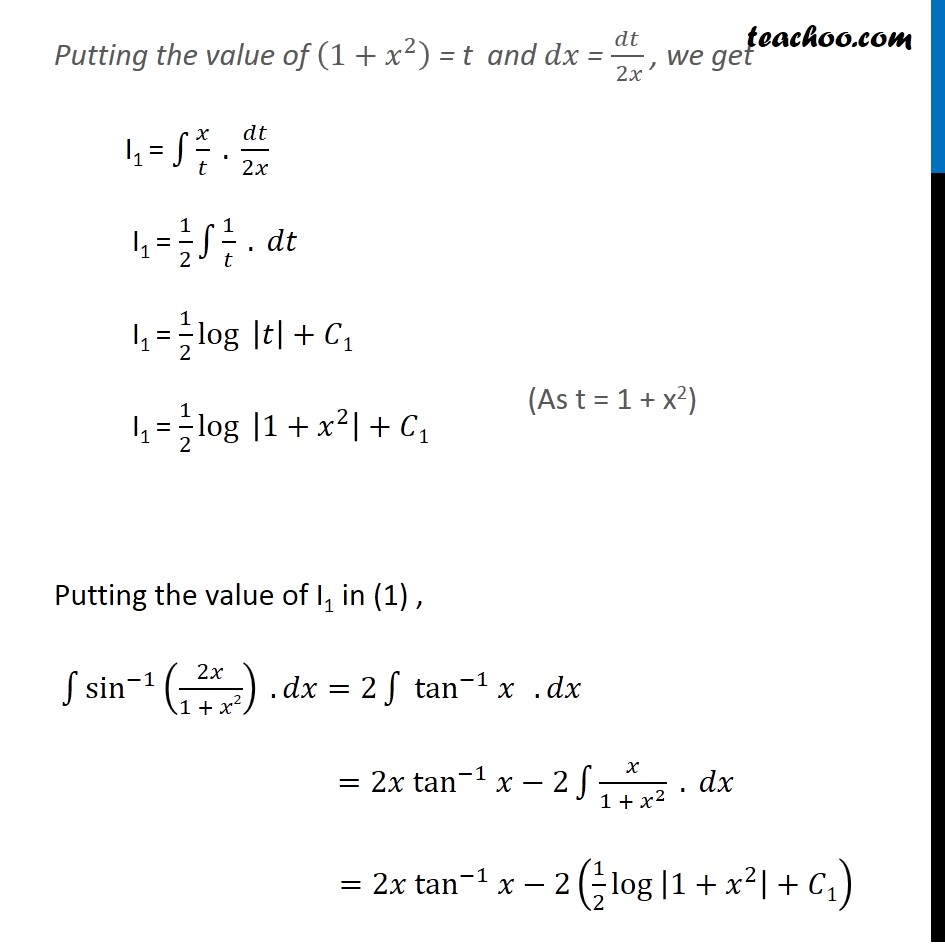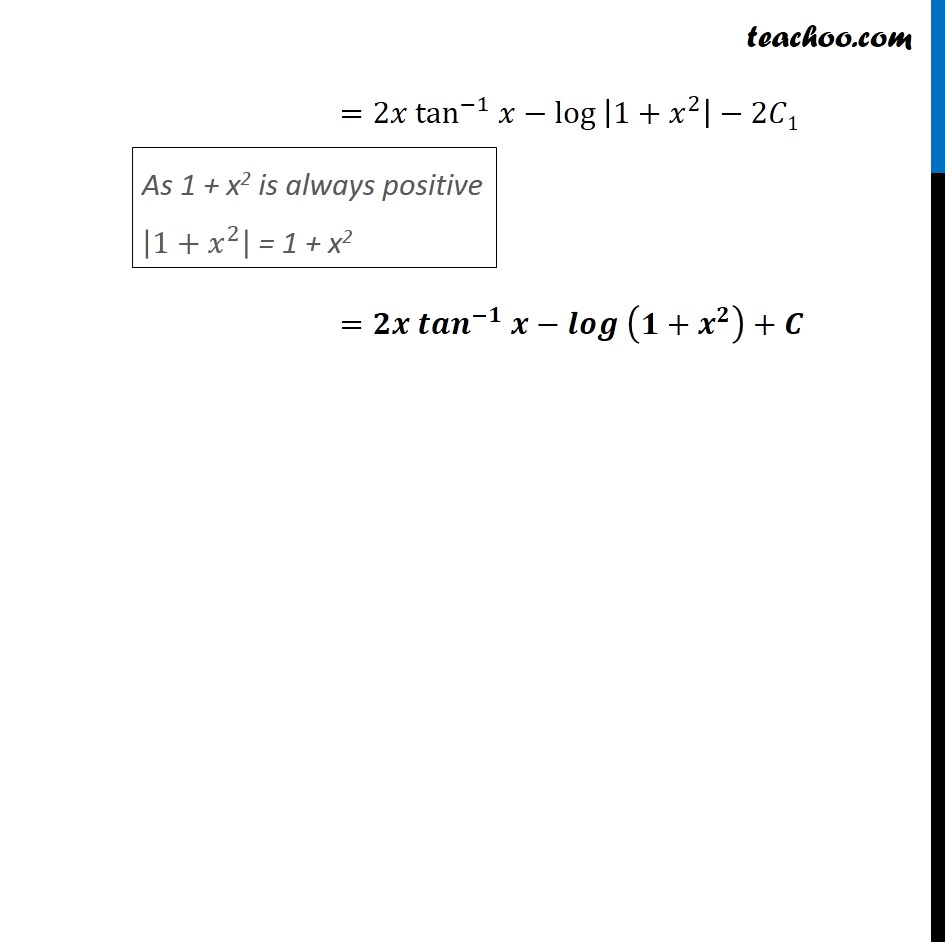Learn in your speed, with individual attention - Teachoo Maths 1-on-1 Class

### Transcript

Ex 7.6, 22 Integrate the function sin^(−1) (2𝑥/(1 + 𝑥2)) Simplifying the given function sin^(−1) (2𝑥/(1 + 𝑥2)) Let 𝑥=tan⁡𝑡 ∴ 𝑡=tan^(−1)⁡(𝑥) ∴ sin^(−1) (2𝑥/(1 + 𝑥2))=sin^(−1) ((2 tan⁡𝑡" " )/(1 + tan^2⁡𝑡 )) =sin^(−1) (sin⁡2𝑡 ) = 2t Ex 7.6, 22 Integrate the function sin^(−1) (2𝑥/(1 + 𝑥2)) Simplifying the given function sin^(−1) (2𝑥/(1 + 𝑥2)) Let 𝑥=tan⁡𝑡 ∴ 𝑡=tan^(−1)⁡(𝑥) ∴ sin^(−1) (2𝑥/(1 + 𝑥2))=sin^(−1) ((2 tan⁡𝑡" " )/(1 + tan^2⁡𝑡 )) =sin^(−1) (sin⁡2𝑡 ) = 2t (𝑈𝑠𝑖𝑛𝑔 sin⁡2𝜃=(2 tan⁡𝜃" " )/(1 + tan^2⁡𝜃 )) (𝑈𝑠𝑖𝑛𝑔 sin^(−1) (sin⁡𝑥 )=𝑥) =2 tan^(−1)⁡𝑥 Thus, our function becomes ∫1▒〖sin^(−1) (2𝑥/(1 + 𝑥2)) 𝑑𝑥〗 = 2∫1▒〖tan^(−1)⁡𝑥 𝑑𝑥〗 =2∫1▒〖(tan^(−1) 𝑥) 1.𝑑𝑥 " " 〗 = 2 tan^(−1) 𝑥∫1▒〖1 .〗 𝑑𝑥−2∫1▒(𝑑(tan^(−1)⁡𝑥 )/𝑑𝑥 ∫1▒〖1 .𝑑𝑥〗) 𝑑𝑥 = 2tan^(−1) 𝑥 (𝑥)−2∫1▒1/(1 + 𝑥^2 ) . 𝑥 . 𝑑𝑥 Now we know that ∫1▒〖𝑓(𝑥) 𝑔⁡(𝑥) 〗 𝑑𝑥=𝑓(𝑥) ∫1▒𝑔(𝑥) 𝑑𝑥−∫1▒(𝑓′(𝑥)∫1▒𝑔(𝑥) 𝑑𝑥) 𝑑𝑥 Putting f(x) = tan–1 x and g(x) = 1 (𝑈𝑠𝑖𝑛𝑔 𝑡=tan^(−1)⁡(𝑥) ) = 2𝑥 tan^(−1) 𝑥−2∫1▒𝑥/(1 + 𝑥^2 ) . 𝑑𝑥 Solving I1 I1 = ∫1▒𝑥/(1 + 𝑥^2 ) . 𝑑𝑥" " Let 1 + 𝑥^2=𝑡 Differentiating both sides 𝑤.𝑟.𝑡.𝑥 0 + 2𝑥=𝑑𝑡/𝑑𝑥 𝑑𝑥=𝑑𝑡/2𝑥 Our equation becomes I1 = ∫1▒𝑥/(1 + 𝑥^2 ) . 𝑑𝑥" " Putting the value of (1+𝑥^2 ) = t and 𝑑𝑥 = 𝑑𝑡/( 2𝑥) , we get I1 = ∫1▒𝑥/𝑡 . 𝑑𝑡/2𝑥 I1 = 1/2 ∫1▒1/𝑡 . 𝑑𝑡 I1 = 1/2 log⁡〖 |𝑡|〗+𝐶1 I1 = 1/2 log⁡〖 |1+𝑥^2 |〗+𝐶1 Putting the value of I1 in (1) , ∫1▒〖sin^(−1) (2𝑥/(1 + 𝑥2)) 〗 .𝑑𝑥=2∫1▒〖" " tan^(−1) 𝑥" " 〗 .𝑑𝑥 =2𝑥 tan^(−1) 𝑥−2∫1▒𝑥/(1 + 𝑥^2 ) . 𝑑𝑥 =2𝑥 tan^(−1) 𝑥−2(1/2 〖log 〗⁡|1+𝑥^2 |+𝐶1) (As t = 1 + x2) =2𝑥 tan^(−1) 𝑥−〖log 〗⁡|1+𝑥^2 |−2𝐶1 =𝟐𝒙 〖𝒕𝒂𝒏〗^(−𝟏) 𝒙−〖𝒍𝒐𝒈 〗⁡(𝟏+𝒙^𝟐 )+𝑪 As 1 + x2 is always positive |1+𝑥^2 | = 1 + x2